Acceleration is the rate at which speed increases or decreases.  Acceleration involves a changes in speed or a change in velocity during a time interval. Acceleration can be both a scalar quantity and a vector quantity.  It is a scalar quantity when we consider only its magnitude.  If we also indicate the direction in which a moving object is accelerating, then we are defining acceleration as a vector quantity.

When an object slows down we say it is decelerating. Deceleration (many teachers don't like to use this term) is really negative acceleration.  The symbol for acceleration is  a .  The units for acceleration are [m/s] {"meters-per-second-squared"}."a" with no arrow on top = scalar acceleration;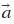(with the arrow on top) = vector acceleration

Acceleration over a definite  time interval is known as average acceleration.  Acceleration calculated at each instant (very small time segment) along the  path of motion of a moving object is known as instantaneous acceleration.

The equation for average acceleration is :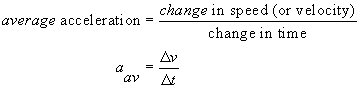Note that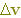is the change in speed or velocity during the time interval (change in time)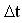.  This means that if at time t1 the speed of an object was v1, at a later time t2, its corresponding speed is v2.[Tip: The symbol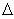("delta")  is a mathematica operatpor that indicates  "a Final quantity  - an Initial quantity".[Your textbook or teacher may use the subscripts "i" for initial and "f"" for final ... this site uses "1" for initial and "2" for final]Example:

A police car is cruising at 50 km/h along a straight road.  A motorist zooms past the cruiser. The police officer steps on the gas and catches up to the speeding car 30 s later at that instant her speedometer was reading 80 km/h.

What was the average acceleration of the police car during the 30 s?

Given:  v1 = 50 km/h   v2 = 80 km/h
let the initial time t = 0  then, t 2 = 30s

Find:  aav

Solution: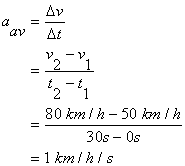The standard unit for acceleration is the m/s2 .  Therefore we will convert our answer () to m/s2 .

1 km = 1000 m   and 1 h = 3600 s   Therefore:The police cruiser accelerated at 0.28 m/s2